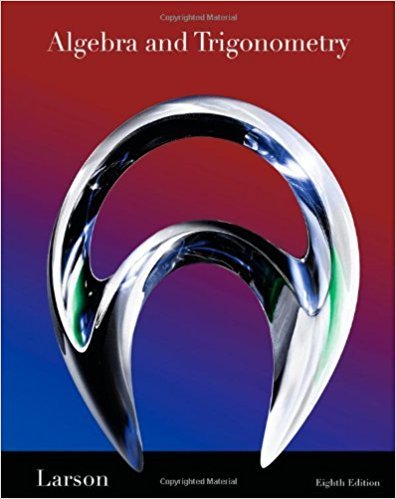×
×

# Solutions for Chapter 2.1: Linear Equations in Two Variables## Full solutions for Algebra and Trigonometry | 8th Edition

ISBN: 9781439048474Solutions for Chapter 2.1: Linear Equations in Two Variables

Solutions for Chapter 2.1
4 5 0 294 Reviews
29
5
##### ISBN: 9781439048474

Chapter 2.1: Linear Equations in Two Variables includes 146 full step-by-step solutions. Algebra and Trigonometry was written by and is associated to the ISBN: 9781439048474. This textbook survival guide was created for the textbook: Algebra and Trigonometry, edition: 8. This expansive textbook survival guide covers the following chapters and their solutions. Since 146 problems in chapter 2.1: Linear Equations in Two Variables have been answered, more than 52007 students have viewed full step-by-step solutions from this chapter.

Key Math Terms and definitions covered in this textbook
• Adjacency matrix of a graph.

Square matrix with aij = 1 when there is an edge from node i to node j; otherwise aij = O. A = AT when edges go both ways (undirected). Adjacency matrix of a graph. Square matrix with aij = 1 when there is an edge from node i to node j; otherwise aij = O. A = AT when edges go both ways (undirected).

• Block matrix.

A matrix can be partitioned into matrix blocks, by cuts between rows and/or between columns. Block multiplication ofAB is allowed if the block shapes permit.

• Condition number

cond(A) = c(A) = IIAIlIIA-III = amaxlamin. In Ax = b, the relative change Ilox III Ilx II is less than cond(A) times the relative change Ilob III lib II· Condition numbers measure the sensitivity of the output to change in the input.

• Elimination.

A sequence of row operations that reduces A to an upper triangular U or to the reduced form R = rref(A). Then A = LU with multipliers eO in L, or P A = L U with row exchanges in P, or E A = R with an invertible E.

• Fourier matrix F.

Entries Fjk = e21Cijk/n give orthogonal columns FT F = nI. Then y = Fe is the (inverse) Discrete Fourier Transform Y j = L cke21Cijk/n.

• Graph G.

Set of n nodes connected pairwise by m edges. A complete graph has all n(n - 1)/2 edges between nodes. A tree has only n - 1 edges and no closed loops.

• Multiplication Ax

= Xl (column 1) + ... + xn(column n) = combination of columns.

• Orthogonal subspaces.

Every v in V is orthogonal to every w in W.

• Pascal matrix

Ps = pascal(n) = the symmetric matrix with binomial entries (i1~;2). Ps = PL Pu all contain Pascal's triangle with det = 1 (see Pascal in the index).

• Positive definite matrix A.

Symmetric matrix with positive eigenvalues and positive pivots. Definition: x T Ax > 0 unless x = O. Then A = LDLT with diag(D» O.

• Random matrix rand(n) or randn(n).

MATLAB creates a matrix with random entries, uniformly distributed on [0 1] for rand and standard normal distribution for randn.

• Rayleigh quotient q (x) = X T Ax I x T x for symmetric A: Amin < q (x) < Amax.

Those extremes are reached at the eigenvectors x for Amin(A) and Amax(A).

• Right inverse A+.

If A has full row rank m, then A+ = AT(AAT)-l has AA+ = 1m.

• Schwarz inequality

Iv·wl < IIvll IIwll.Then IvTAwl2 < (vT Av)(wT Aw) for pos def A.

• Skew-symmetric matrix K.

The transpose is -K, since Kij = -Kji. Eigenvalues are pure imaginary, eigenvectors are orthogonal, eKt is an orthogonal matrix.

• Solvable system Ax = b.

The right side b is in the column space of A.

• Special solutions to As = O.

One free variable is Si = 1, other free variables = o.

• Triangle inequality II u + v II < II u II + II v II.

For matrix norms II A + B II < II A II + II B II·

• Tridiagonal matrix T: tij = 0 if Ii - j I > 1.

T- 1 has rank 1 above and below diagonal.

• Wavelets Wjk(t).

Stretch and shift the time axis to create Wjk(t) = woo(2j t - k).

×